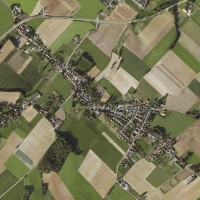Welcome to Bingelder.nl Photography - OtherHome   - Home Mardi Gras Indians   - MGI Calendar Photography Hobby Links Recent changes Contact Hi Guys, welcome to the all-new Bingelder.nl   Other photography. Carnaval in Bengelder, february 10, 2018 Back to other photography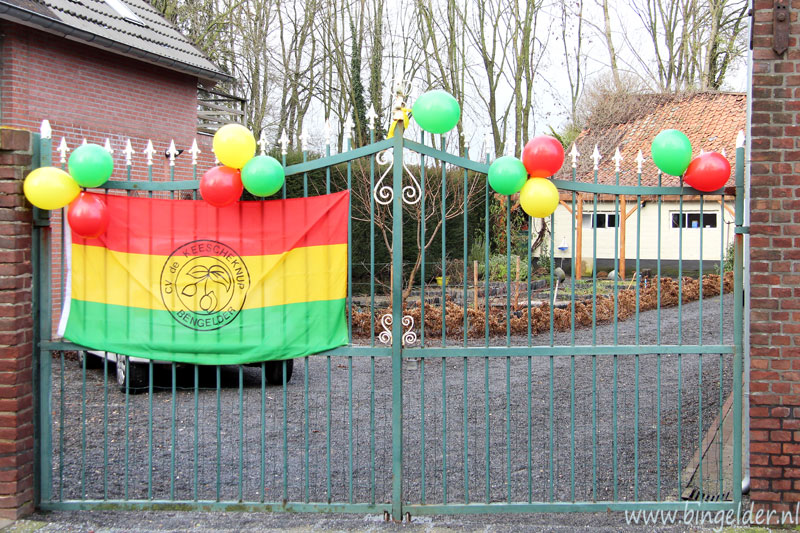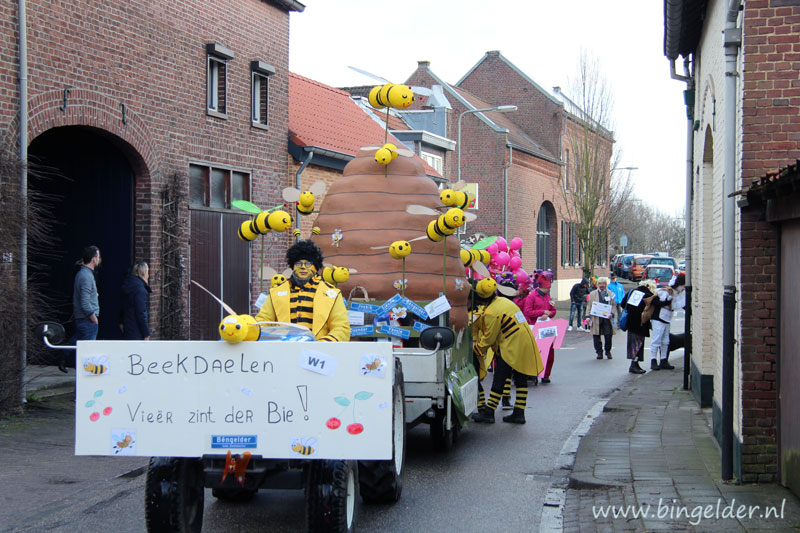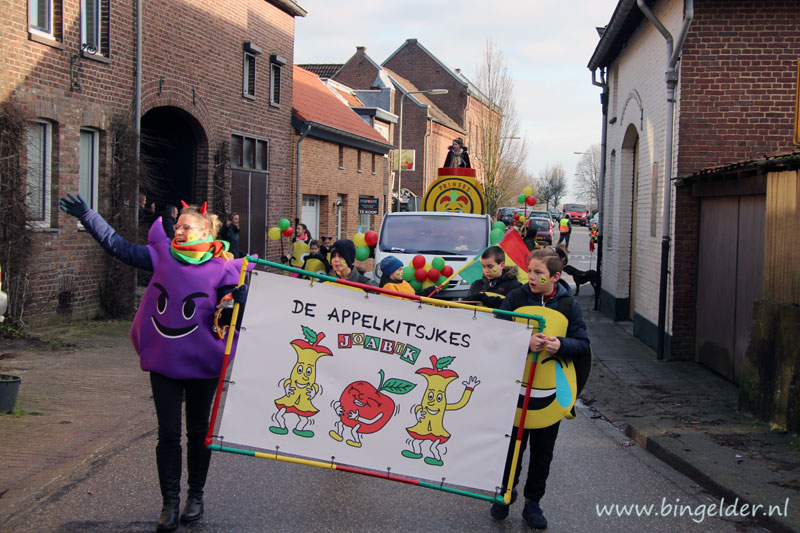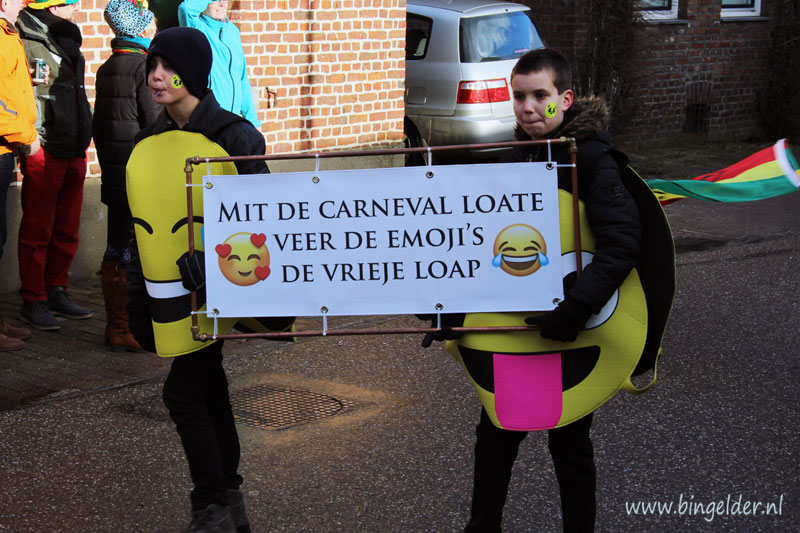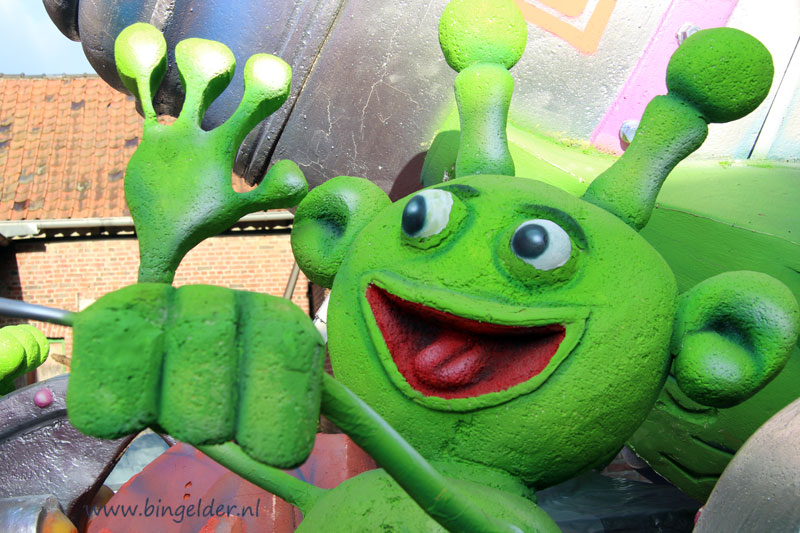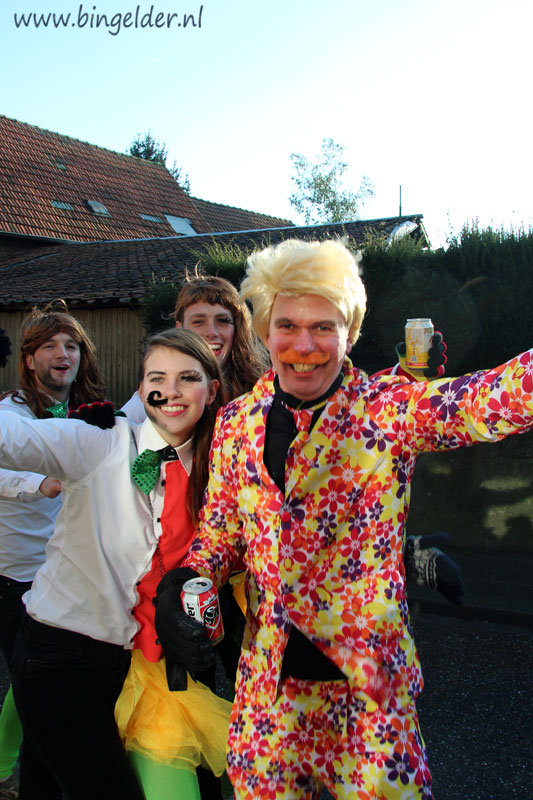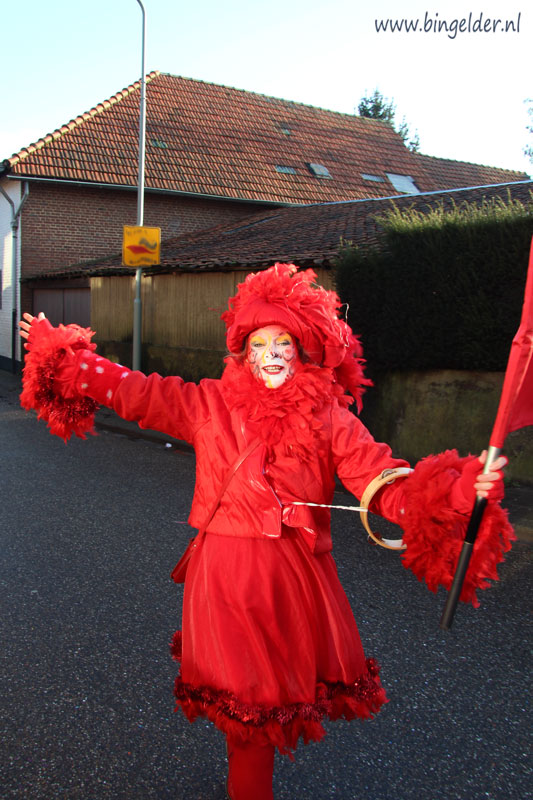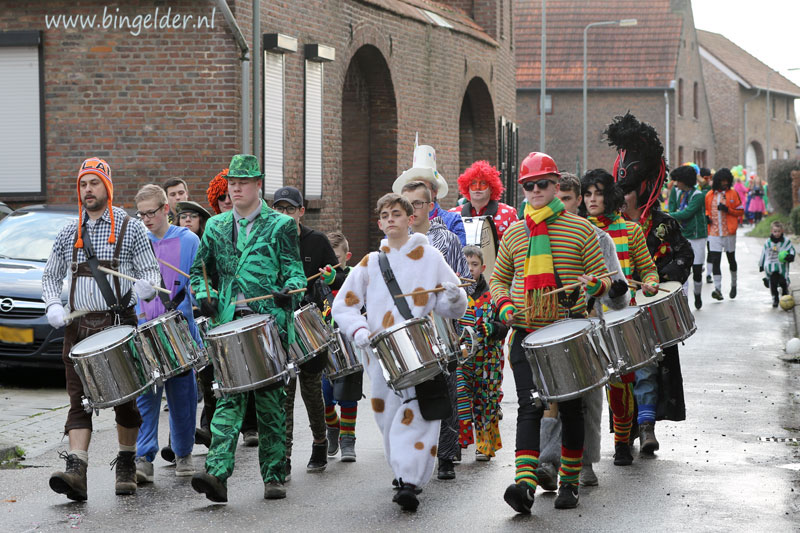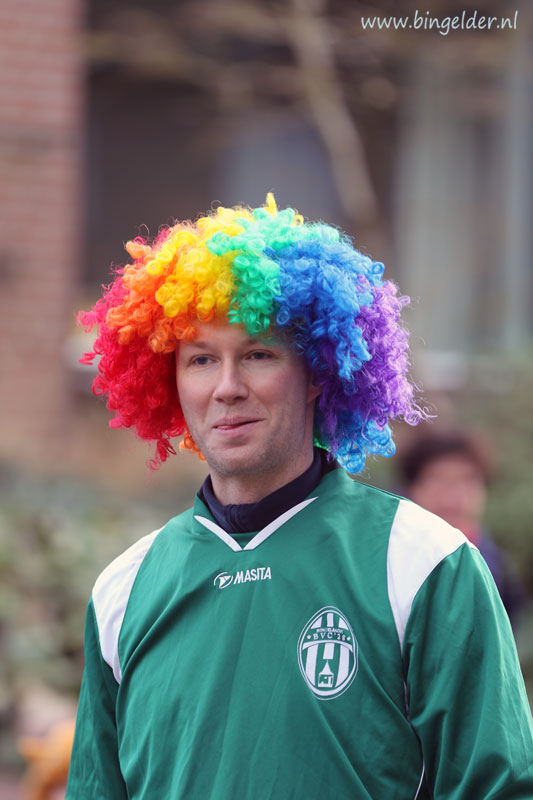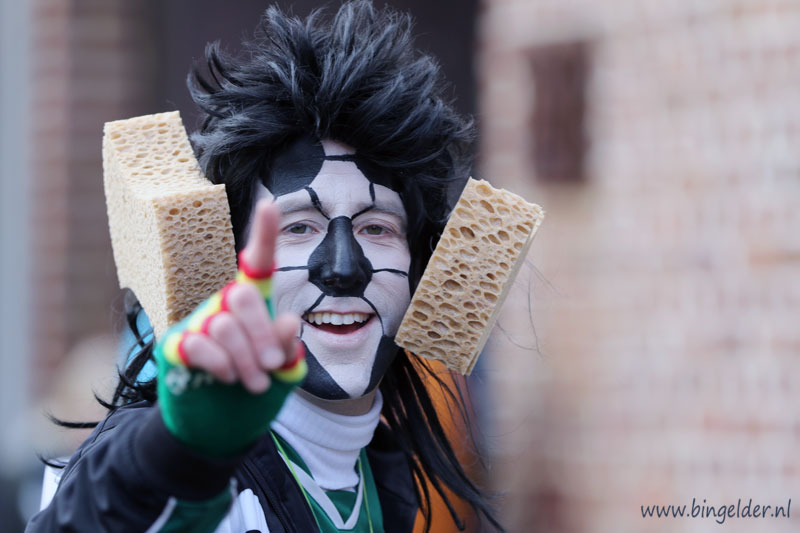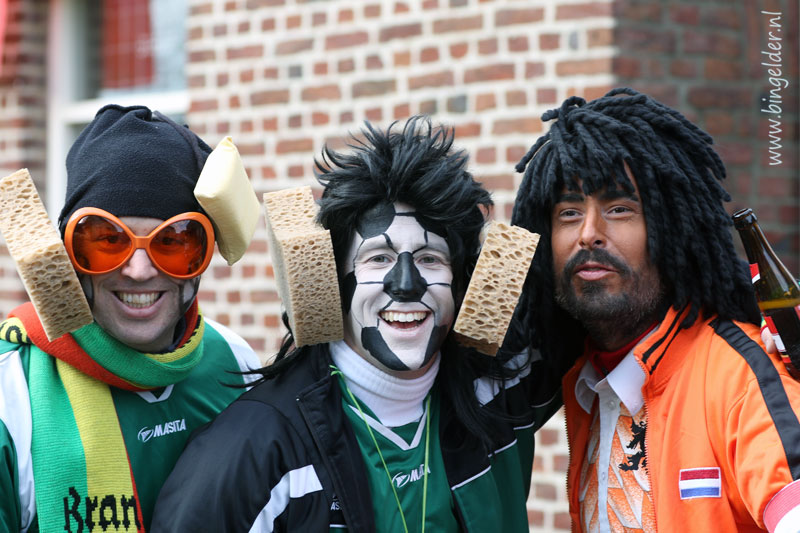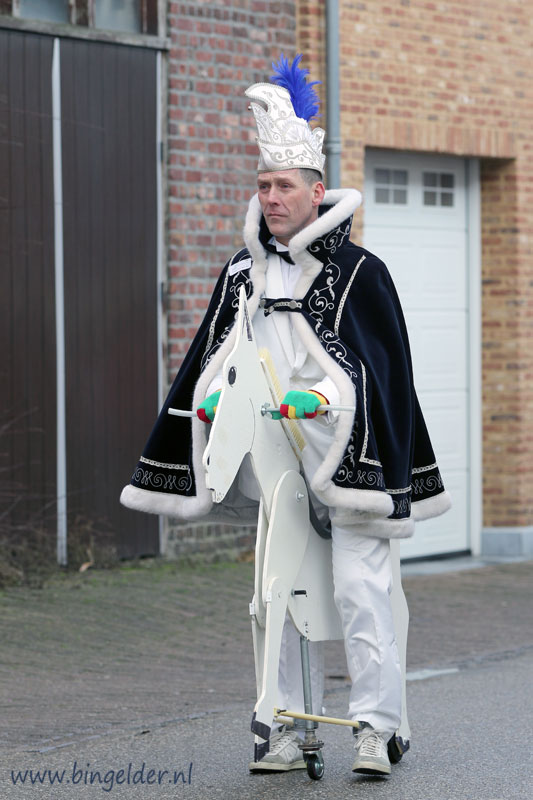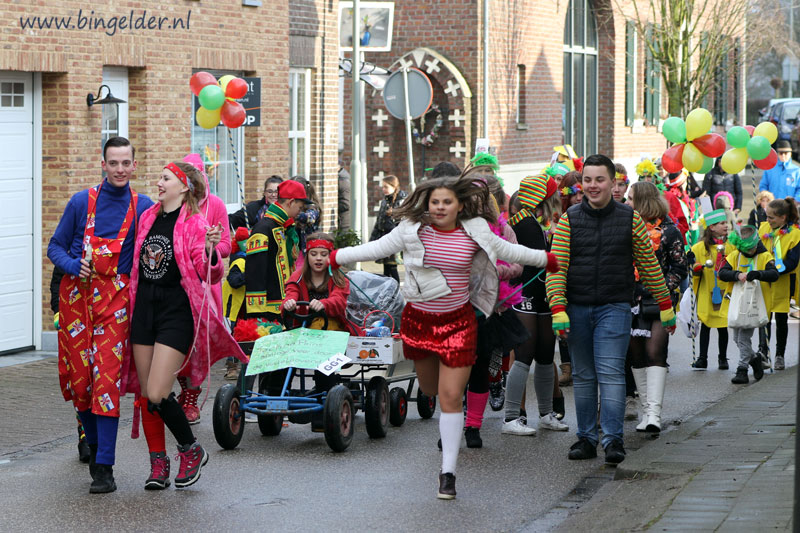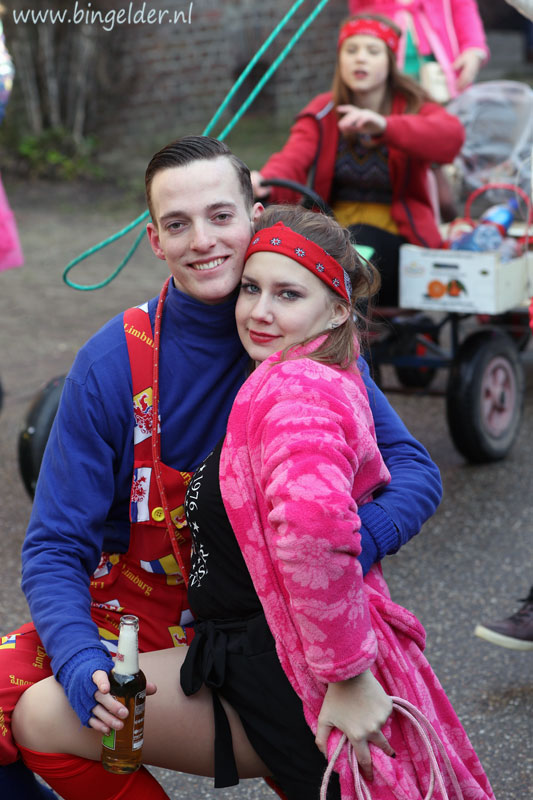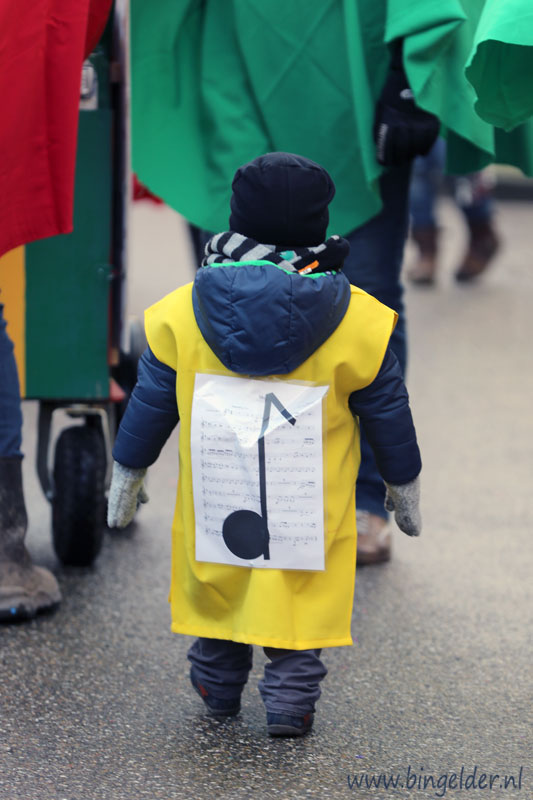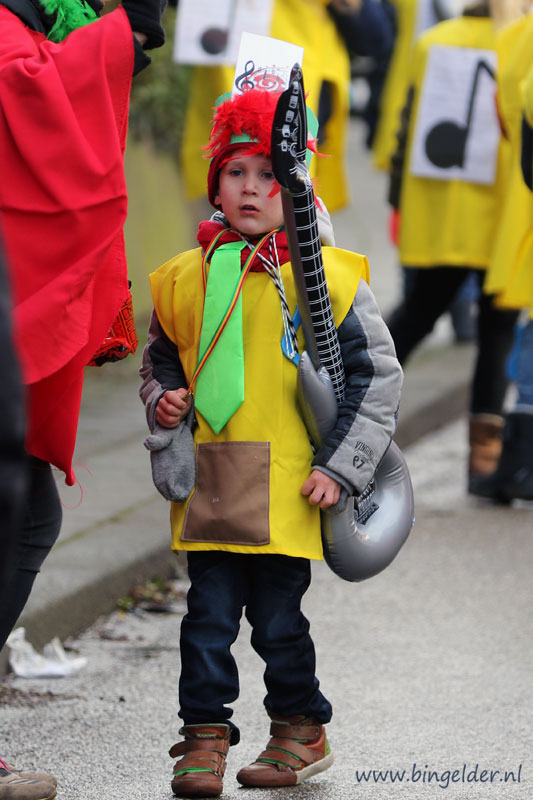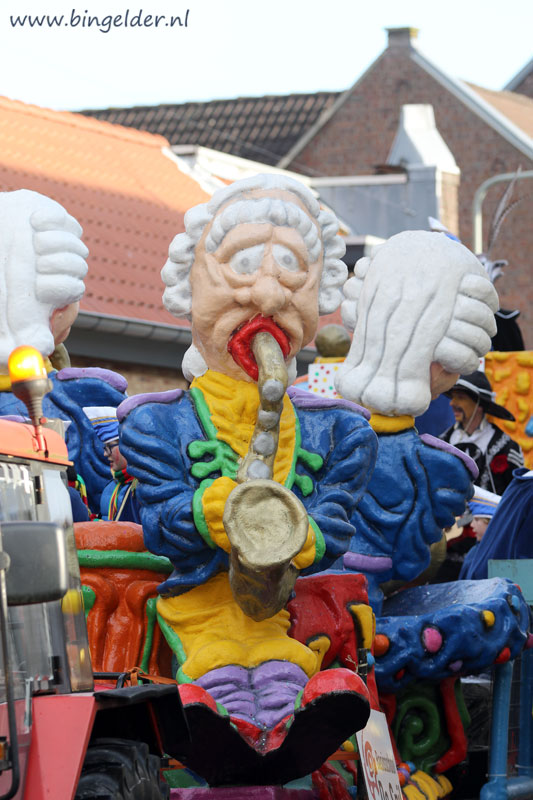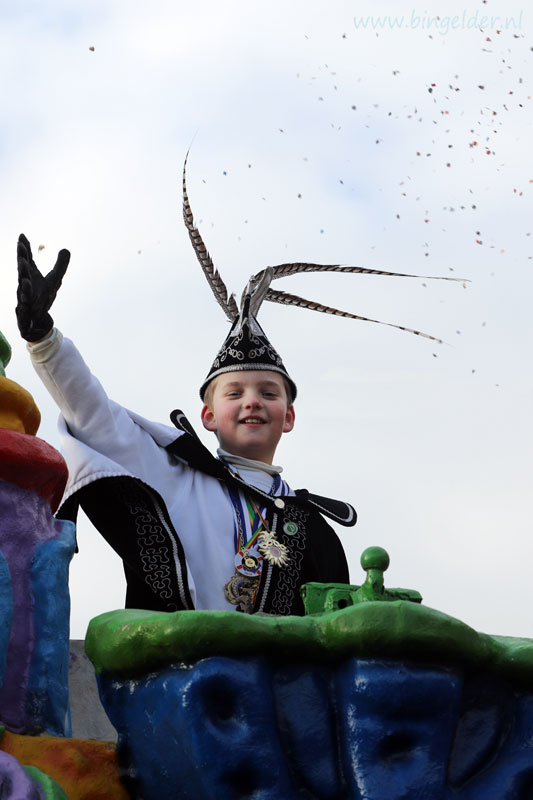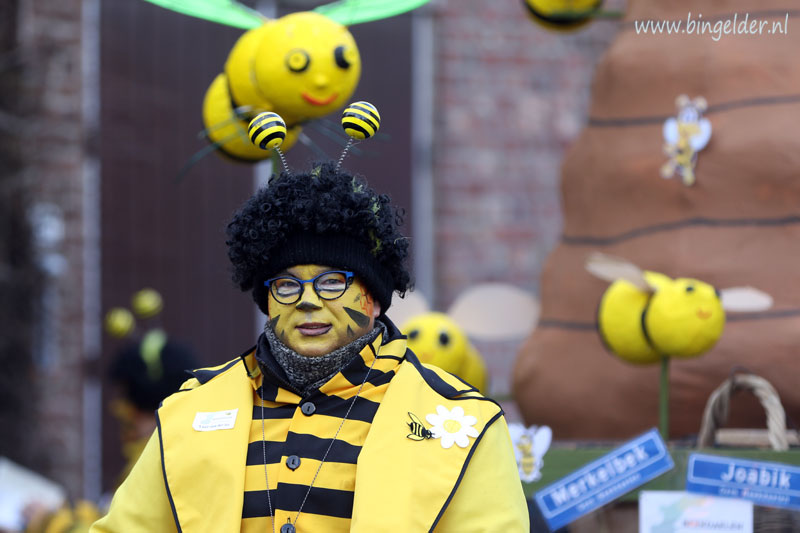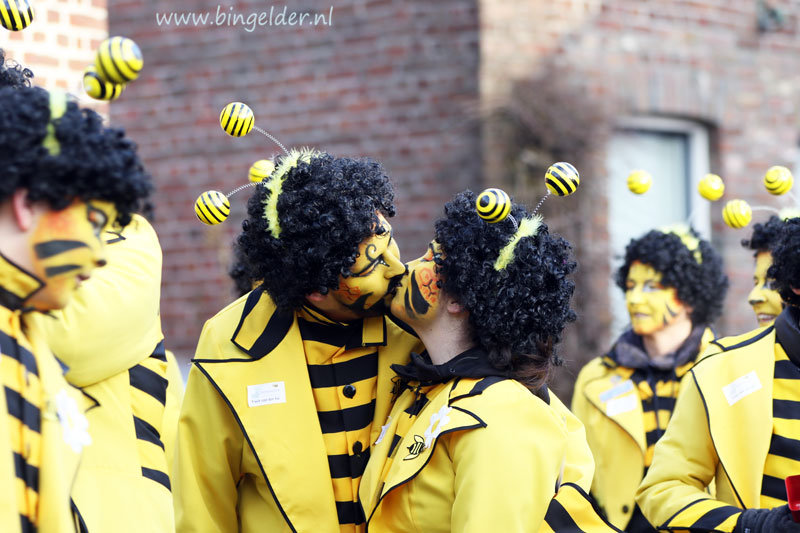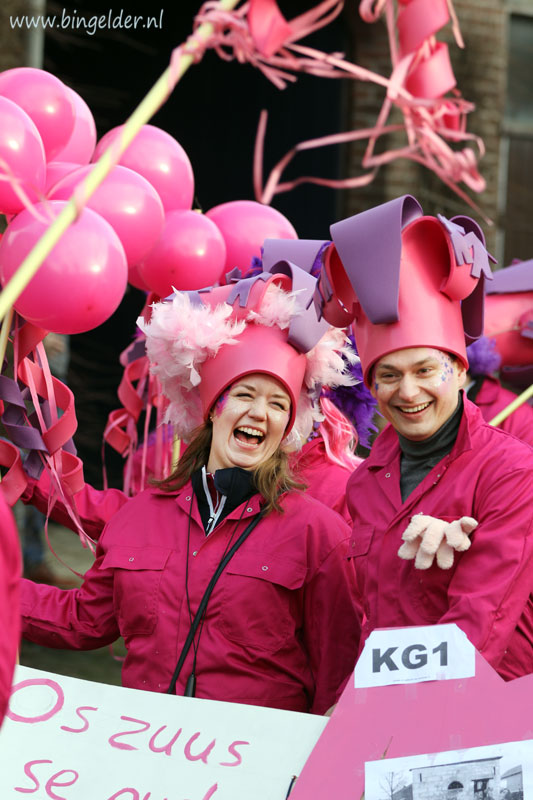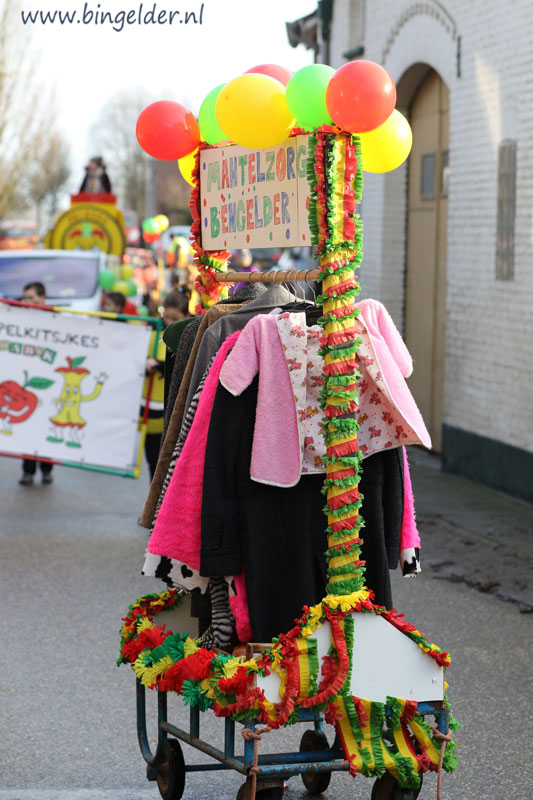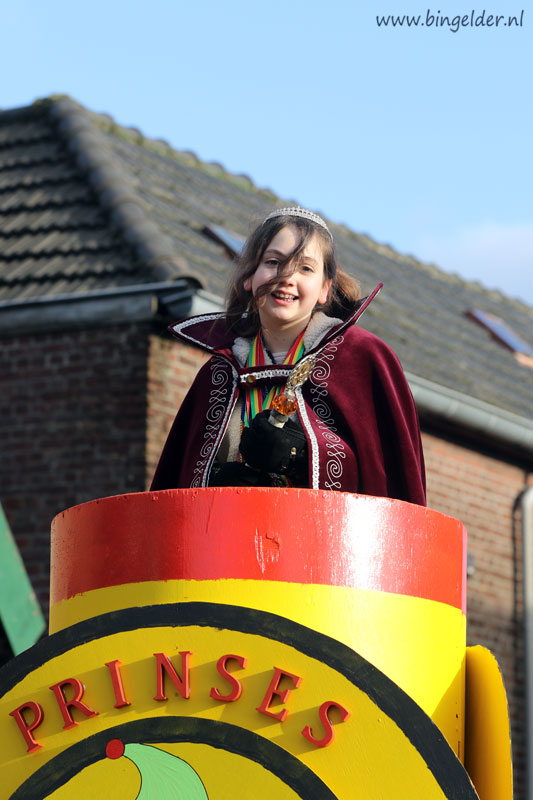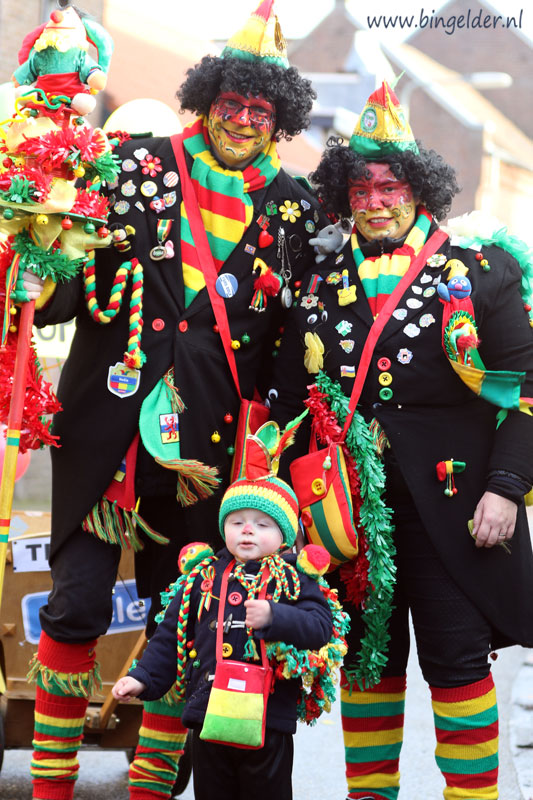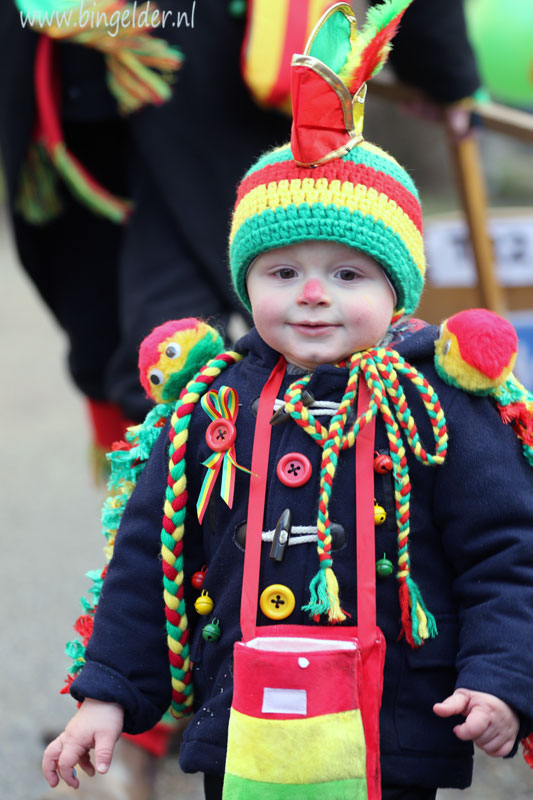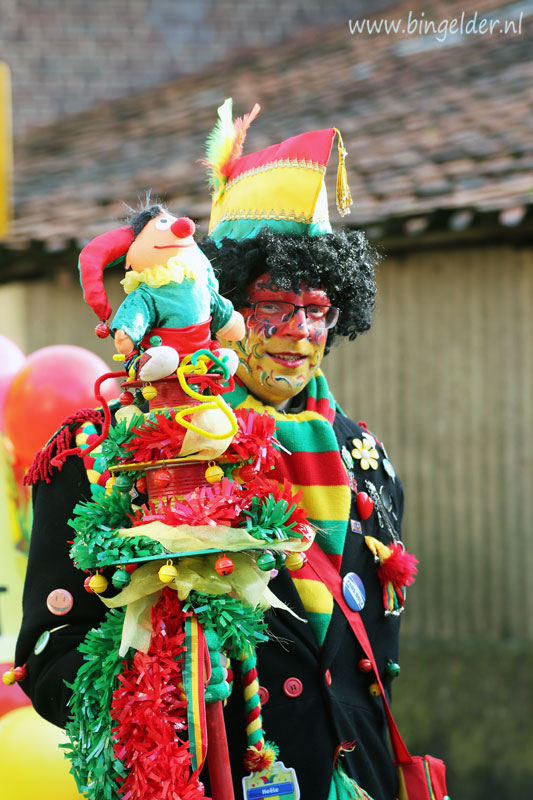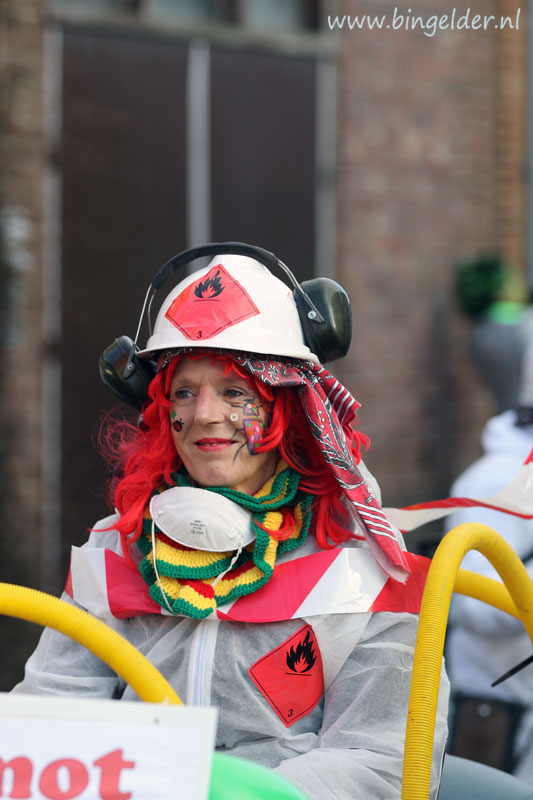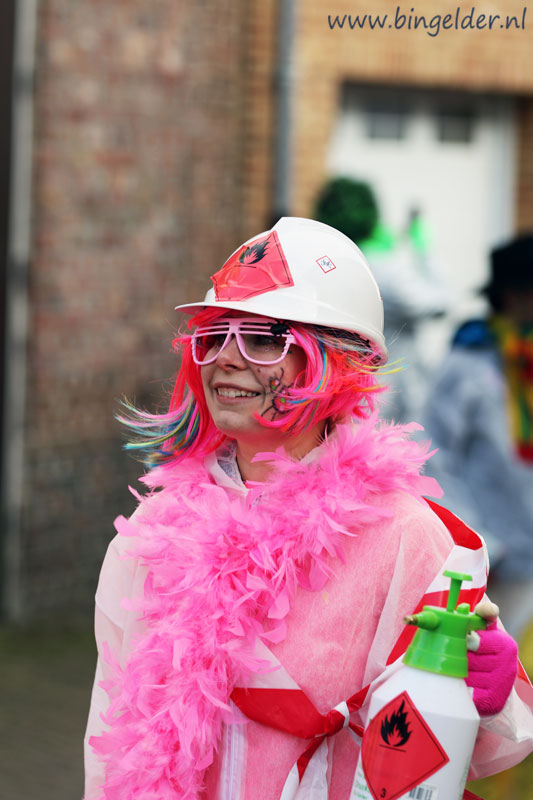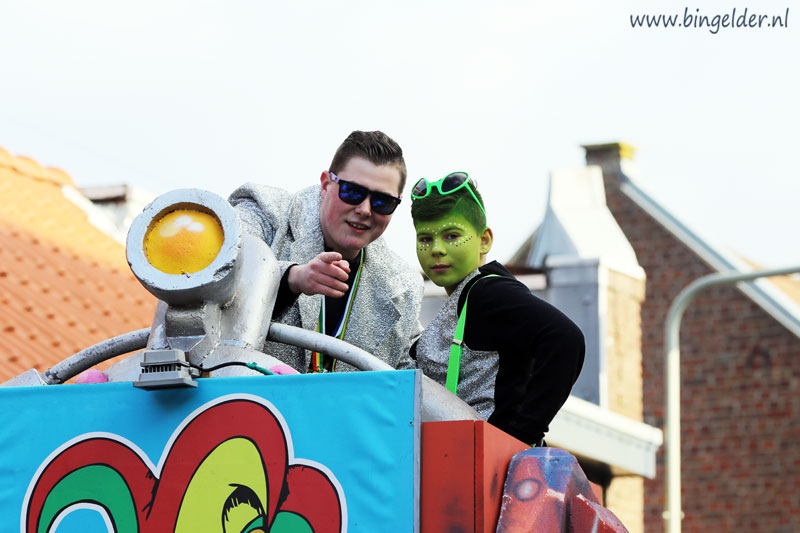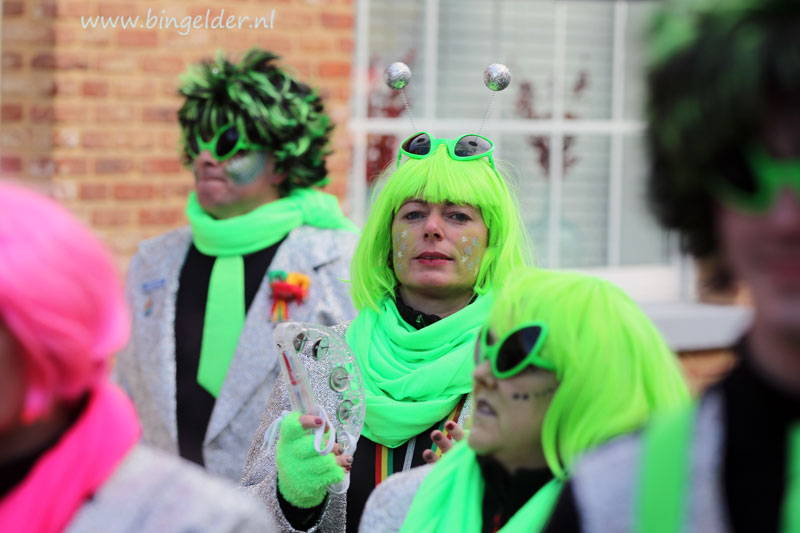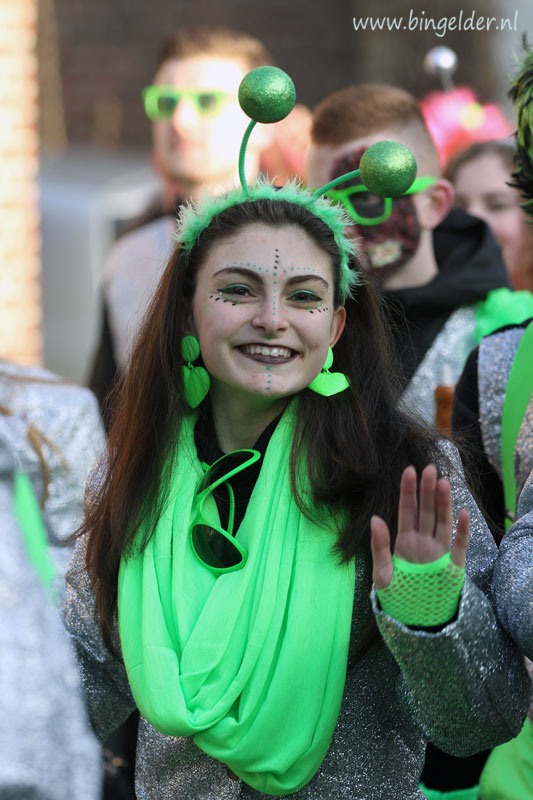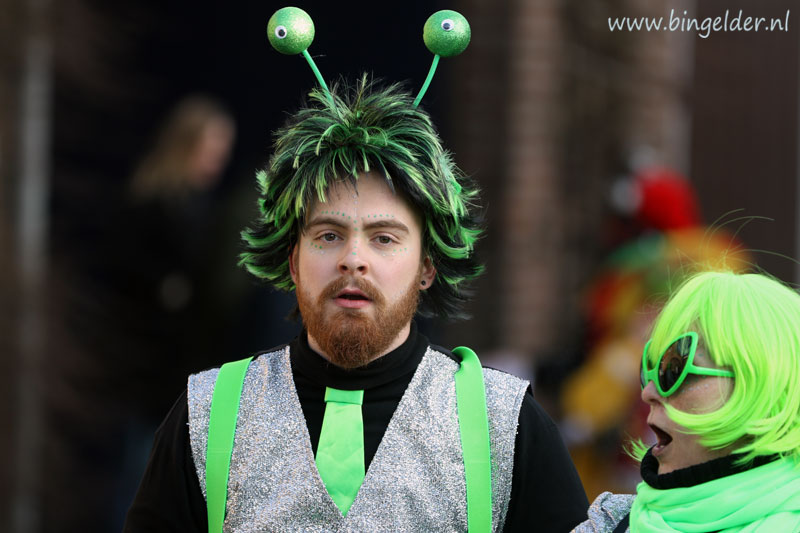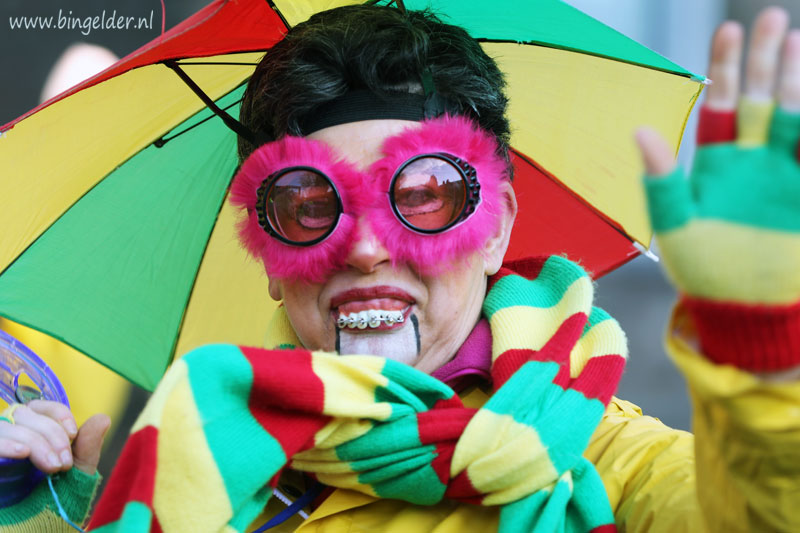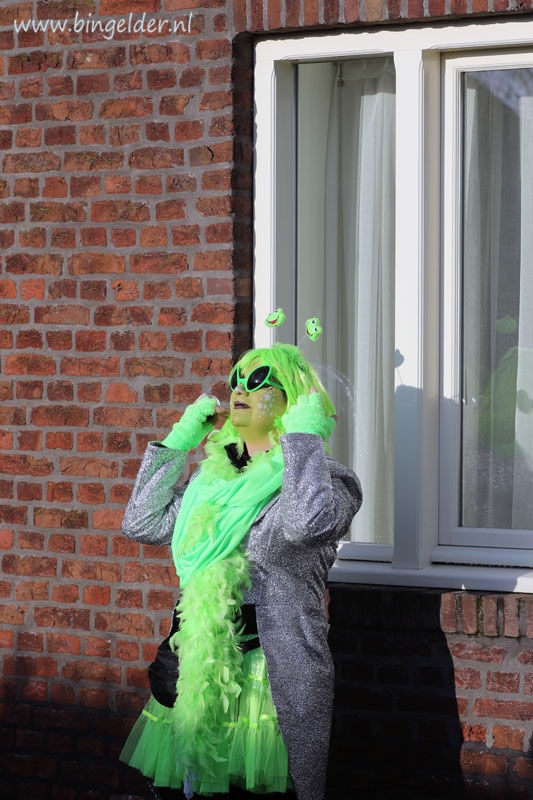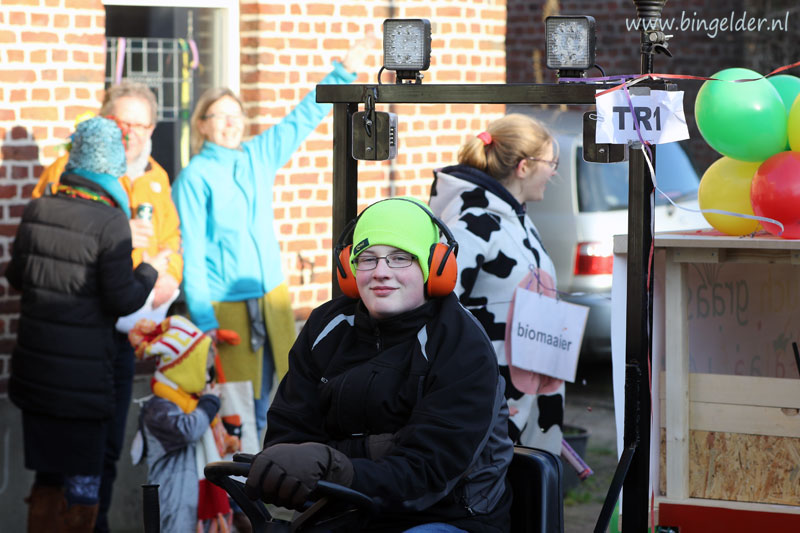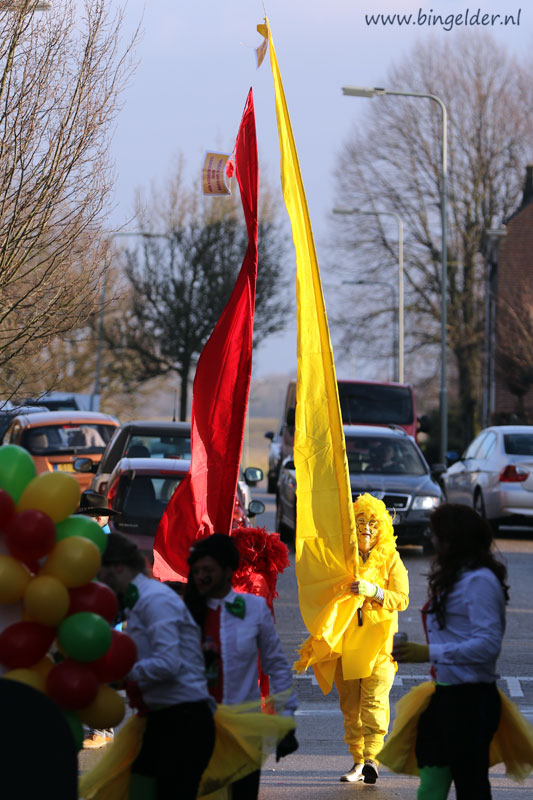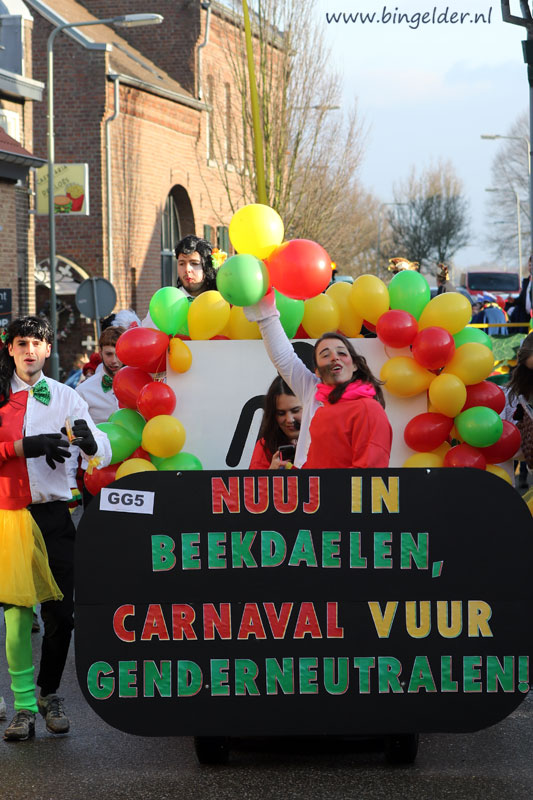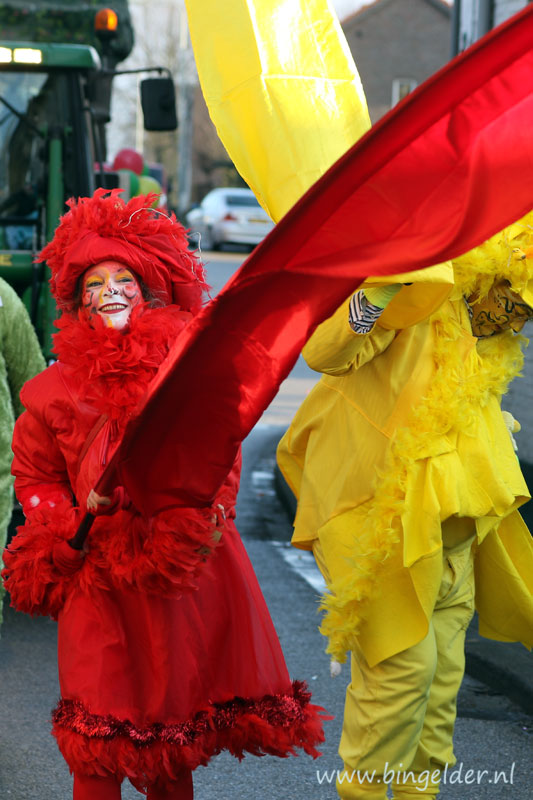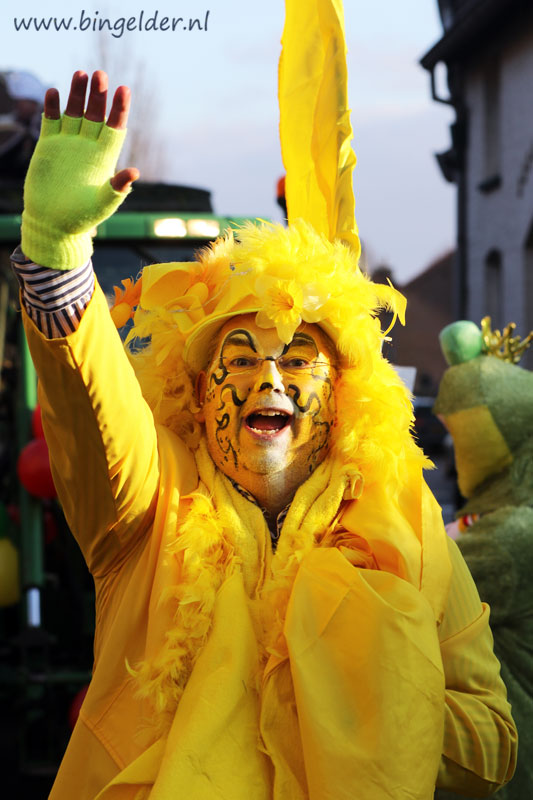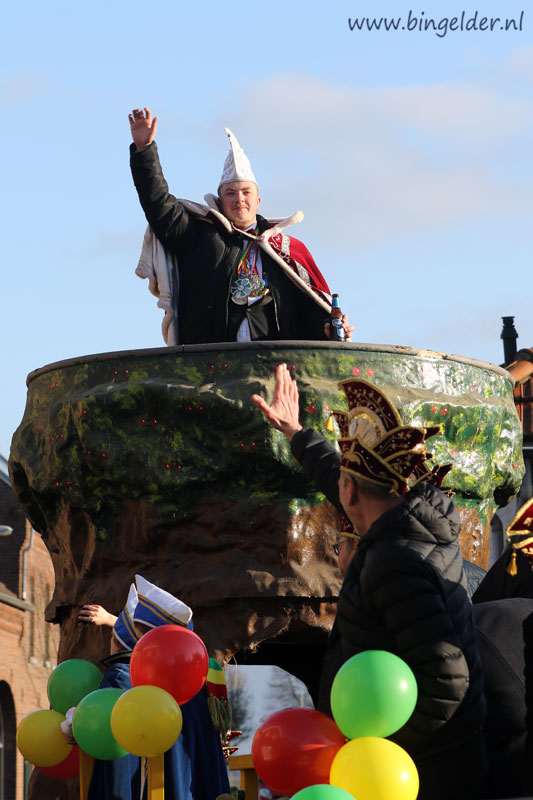Hoie! Tot volgend jaar! P H O T O G R A P H Y - O T H E R P H O T O G R A P H Y - O T H E R P H O T O G R A P H Y - O T H E R P H O T O G R A P H Y - O T H E R P H O T O G R A P H Y - O T H E R P H O T O G R A P H Y - O T H E R P H O T O G R A P H Y - O T H E R P H O T O G R A P H Y - O T H E R P H O T O G R A P H Y - O T H E R P H O T O G R A P H Y - O T H E R P H O T O G R A P H Y - O T H E R P H O T O G R A P H Y - O T H E R P H O T O G R A P H Y - O T H E R P H O T O G R A P H Y - O T H E R P H O T O G R A P H Y - O T H E R P H O T O G R A P H Y - O T H E R P H O T O G R A P H Y - O T H E RThis site created with KompoZer with additional HTML-editing in Kate